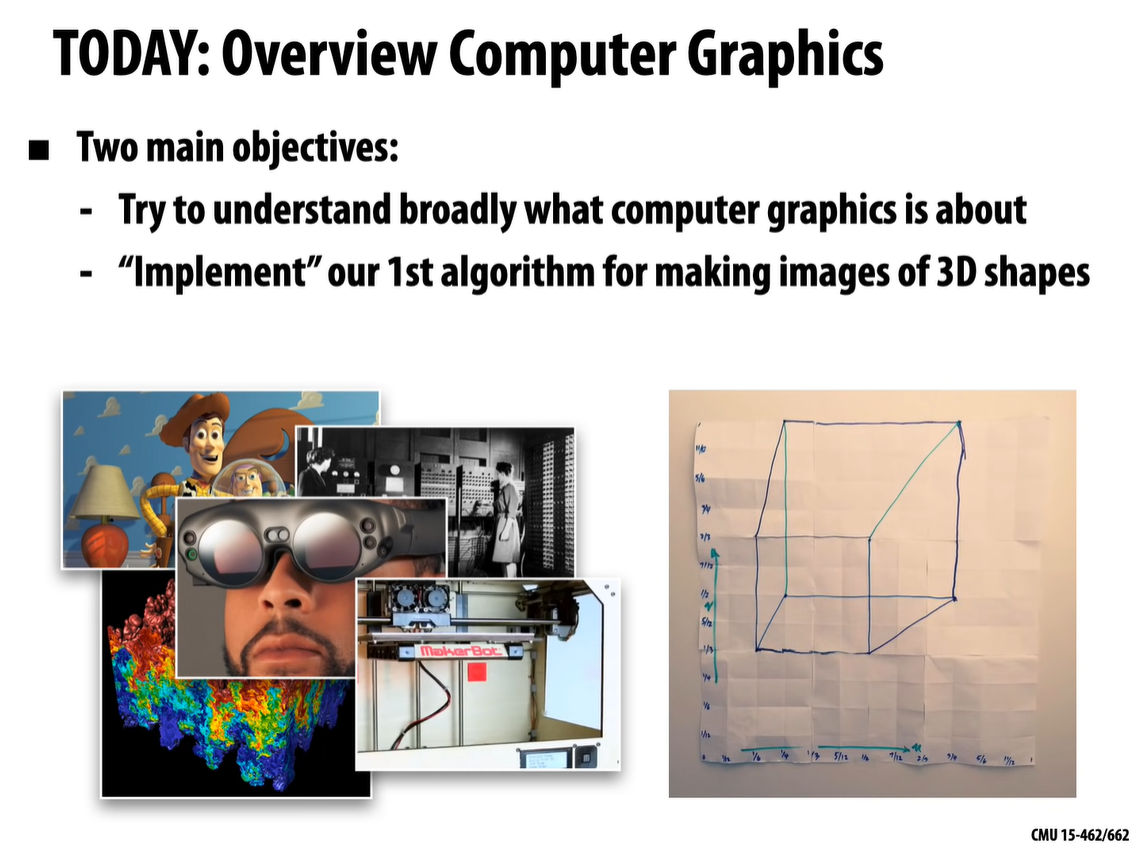The first challenge, to understand a drawing algorithm: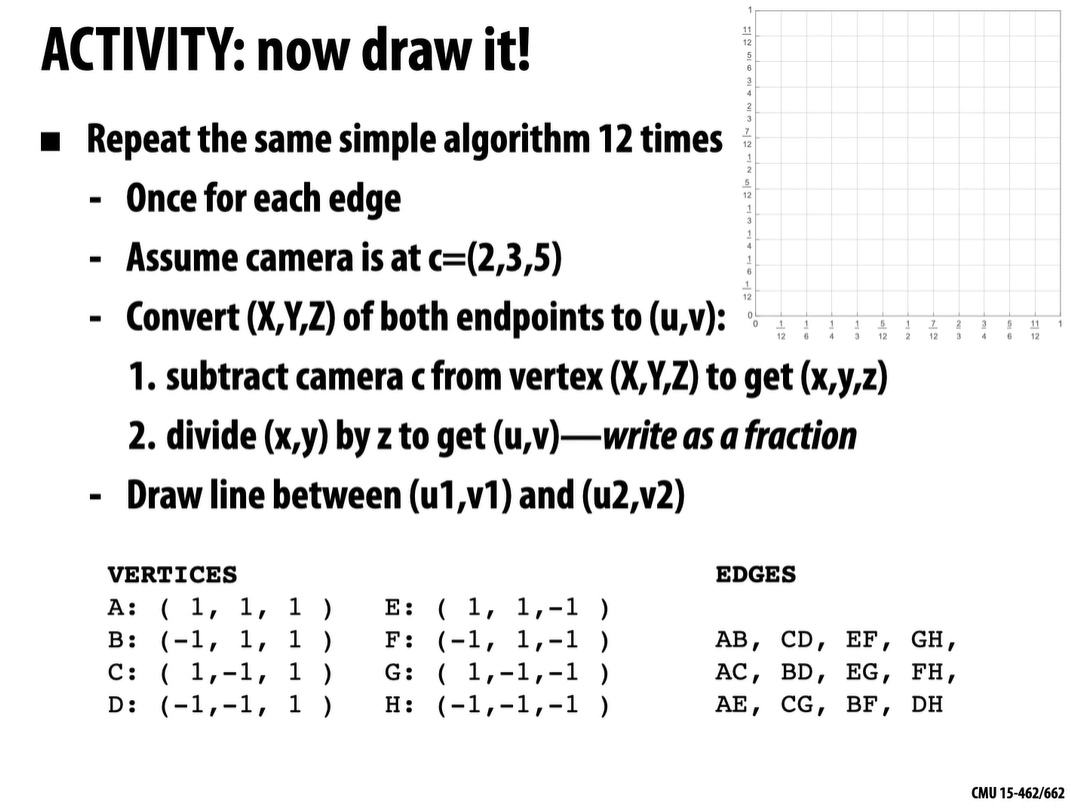The result is supposed to look something like this: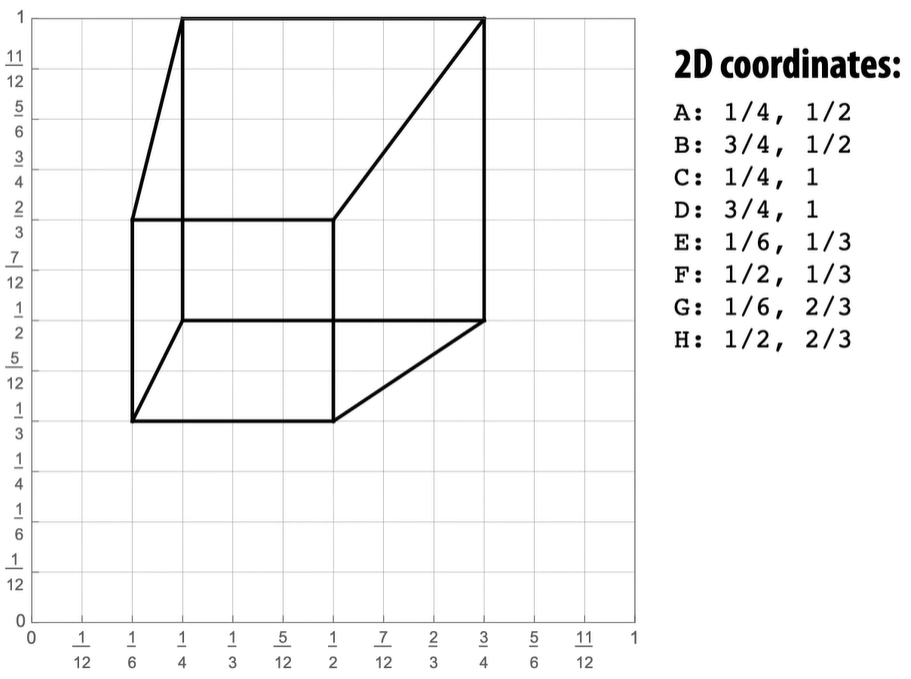My approach in Unity: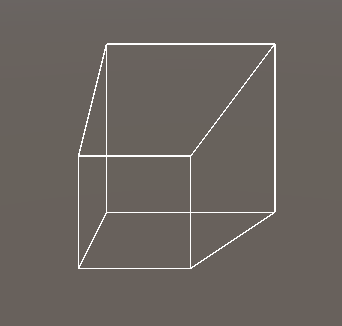Managed to get this with Gizmos.DrawLine(), but it came out inverted for some reason. Also, make sure the camera is set to orthographic and looking back, rotated -180 on Y.

Here's the code:

``````using UnityEngine;

public class LinePlotterCube : MonoBehaviour
{
public Camera mainCam;
public Vector3 camPos;
public Vector3[,] edges;

void Start()
{
camPos = new Vector3(2, 3, 5);
mainCam.transform.position = camPos;

Vector3 A = new(1, 1, 1);
Vector3 B = new(-1, 1, 1);
Vector3 C = new(1, -1, 1);
Vector3 D = new(-1, -1, 1);
Vector3 E = new(1, 1, -1);
Vector3 F = new(-1, 1, -1);
Vector3 G = new(1, -1, -1);
Vector3 H = new(-1, -1, -1);

edges = new Vector3[12,2] {
{ A, B },
{ C, D },
{ E, F },
{ G, H },
{ A, C },
{ B, D },
{ E, G },
{ F, H },
{ A, E },
{ C, G },
{ B, F },
{ D, H }
};
}

private void OnDrawGizmos()
{
for (int i = 0; i < edges.GetLength(0); i++)
{
for (int ii = 0; ii < edges.GetLength(1); ii++)
{
//1- Subtract camera c from vertex
Vector3 uv1 = edges[i, 0] - camPos;
Vector3 uv2 = edges[i, 1] - camPos;
//2 - Divide (x,y) by z to get (u,v)
uv1 = new Vector3(uv1.x / uv1.z, uv1.y / uv1.z, 0);
uv2 = new Vector3(uv2.x / uv2.z, uv2.y / uv2.z, 0);

Gizmos.DrawLine(uv1, uv2);
}
}
}
}

``````

### Rasterization

Color everything touched by the line: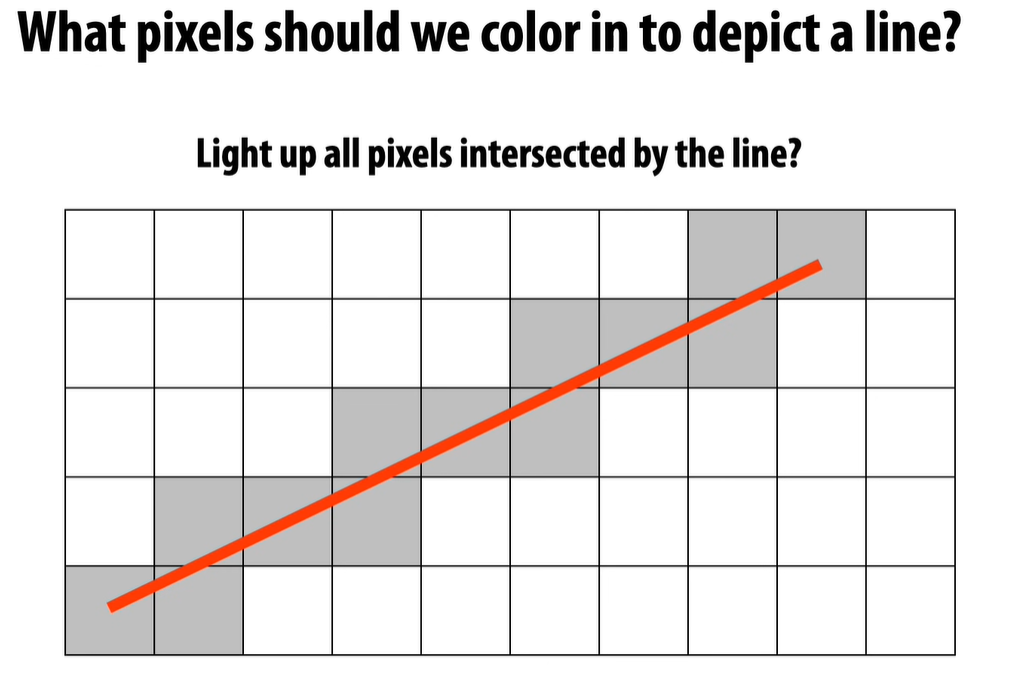Diamond rule: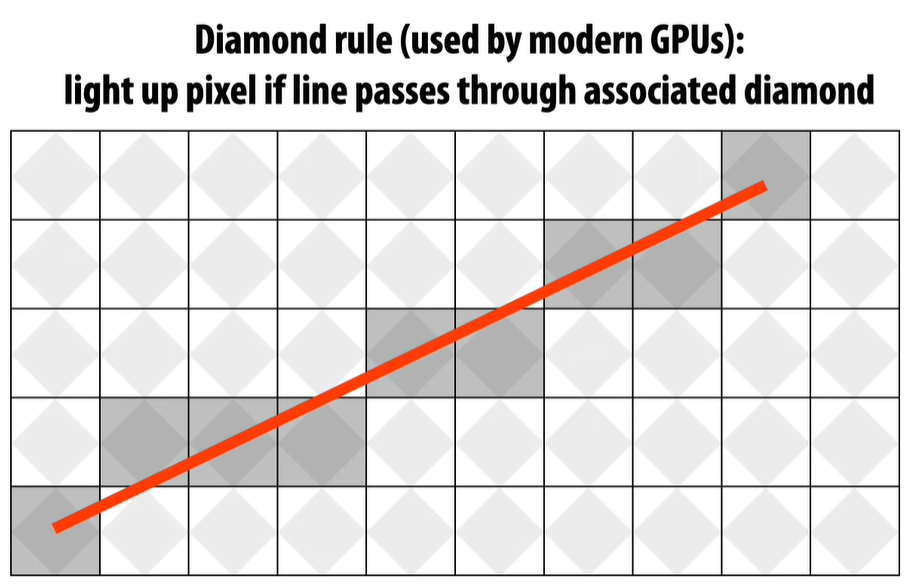Ok, so while talking about rasterization rules, he mentioned O(n) notation. I'm not familiar with this, embarrassingly enough. But that changes now!

### A beginner's guide to Big O Notation

Ok, I've read it. Very elucidating.

That's the end of the lecture. I'm very excited for the next one.